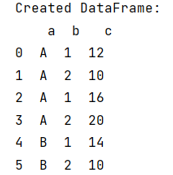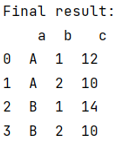# How to get topmost N records within each group of a Pandas DataFrame?

Given a Pandas DataFrame, we have to get topmost N records within each group. By Pranit Sharma Last updated : September 22, 2023

Rows in pandas are the different cell (column) values which are aligned horizontally and also provides uniformity. Each row can have same or different value. Rows are generally marked with the index number but in pandas we can also assign index name according to the needs.

## Problem statement

Given a Pandas DataFrame, we have to get topmost N records within each group.

## Getting topmost N records within each group of a Pandas DataFrame

For this purpose, we will first use pandas.DataFrame.groupby() and then we will select topmost N record by using the following piece of code with the groupby result,

```head(N).reset_index(drop=True)
```

Let us understand with the help of an example,

## Python program to get topmost N records within each group of a Pandas DataFrame

```# Importing pandas package
import pandas as pd

# Create dictionary
d = {
'a':['A','A','A','A','B','B'],
'b':[1,2,1,2,1,2],
'c':[12,10,16,20,14,10]
}

# Create DataFrame
df = pd.DataFrame(d)

# Display DataFrame
print("Created DataFrame:\n",df)

# Groupby function
result = df.groupby('a', as_index=False)

# Selecting 1st row of group by result

# Display final result
print("Final result:\n",final)
```

### Output

The output of the above program is:

Original DataFrame:Topmost N records in groupby result: